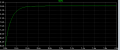# Initial rate of growth of the current

#### Antony 16171

Joined Apr 7, 2021
11
Hello , i was asked to find ''the Initial rate of growth of the current'' but am not quiet sure how do i do that ,

Full questions:
A coil having a resistance of 33ohms and an inductance of 3.3H is connected across a 100v dc supply.

So i used Ltspice to find it ,but am not sure what measurement i need to take .

Edit:i came up with (e/33)*(1-e^(-2/(3.3/33))) = 0.0823721764501894 , is that right ?Last edited:

#### wayneh

Joined Sep 9, 2010
17,405
Ditch the simulator and recall your math. dI/dt at t=0 = ?
Maybe you've got it but I can't check your work if you don't show it.
This is homework, right?

#### Jony130

Joined Feb 17, 2009
5,455
Maybe the initial rate will is equal to L/V = 3.3H/100V = 0.033 s/A

#### Antony 16171

Joined Apr 7, 2021
11
Hello , i was asked to find ''the Initial rate of growth of the current'' but am not quiet sure how do i do that ,

Full questions:
A coil having a resistance of 33ohms and an inductance of 3.3H is connected across a 100v dc supply.

So i used Ltspice to find it ,but am not sure what measurement i need to take .

Edit:i came up with (e/33)*(1-e^(-2/(3.3/33))) = 0.0823721764501894 , is that right ?View attachment 235257
ive done 2 equations but not sure if 1st one or 2nd one is right or none of them at all , i did.
(e/33)*(1-e^(-2/(3.3/33))) = 0.0823721764501894
and
L/V=3.3H/100V=0.033 s/a

#### Antony 16171

Joined Apr 7, 2021
11
Ditch the simulator and recall your math. dI/dt at t=0 = ?
Maybe you've got it but I can't check your work if you don't show it.
This is homework, right?
so to find the initial rate you just do L/V ?

#### Papabravo

Joined Feb 24, 2006
20,369
so to find the initial rate you just do L/V ?
$V\;=\;L\frac{di}{dt}$
You have the fraction upside down

•Antony 16171

#### wayneh

Joined Sep 9, 2010
17,405
Vr = I • R ohm's law
Vi = L • dI/dt definition of an inductor
Vt - Vr - Vi = 0 Kirchoff's law

Vt - I•R = L•dI/dt algebra
dI/dt = (Vt - I•R)/L = V/L at t=0 when I=0

dI/dt = 100/3.3 = 33A/s^2

C'mon guys, you know this stuff and it's not that hard.

#### Papabravo

Joined Feb 24, 2006
20,369
You cans also ask LTspice to plot the derivative of current i, with respect to t. That will give you an initial value which will decay toward zero.

#### Antony 16171

Joined Apr 7, 2021
11
so to find the initial rate you just do L/V ?
so to find the initial rate you just do L/V ?
Vr = I • R ohm's law
Vi = L • dI/dt definition of an inductor
Vt - Vr - Vi = 0 Kirchoff's law

Vt - I•R = L•dI/dt algebra
dI/dt = (Vt - I•R)/L = V/L at t=0 when I=0

dI/dt = 100/3.3 = 33A/s^2

C'mon guys, you know this stuff and it's not that hard.
Thank you